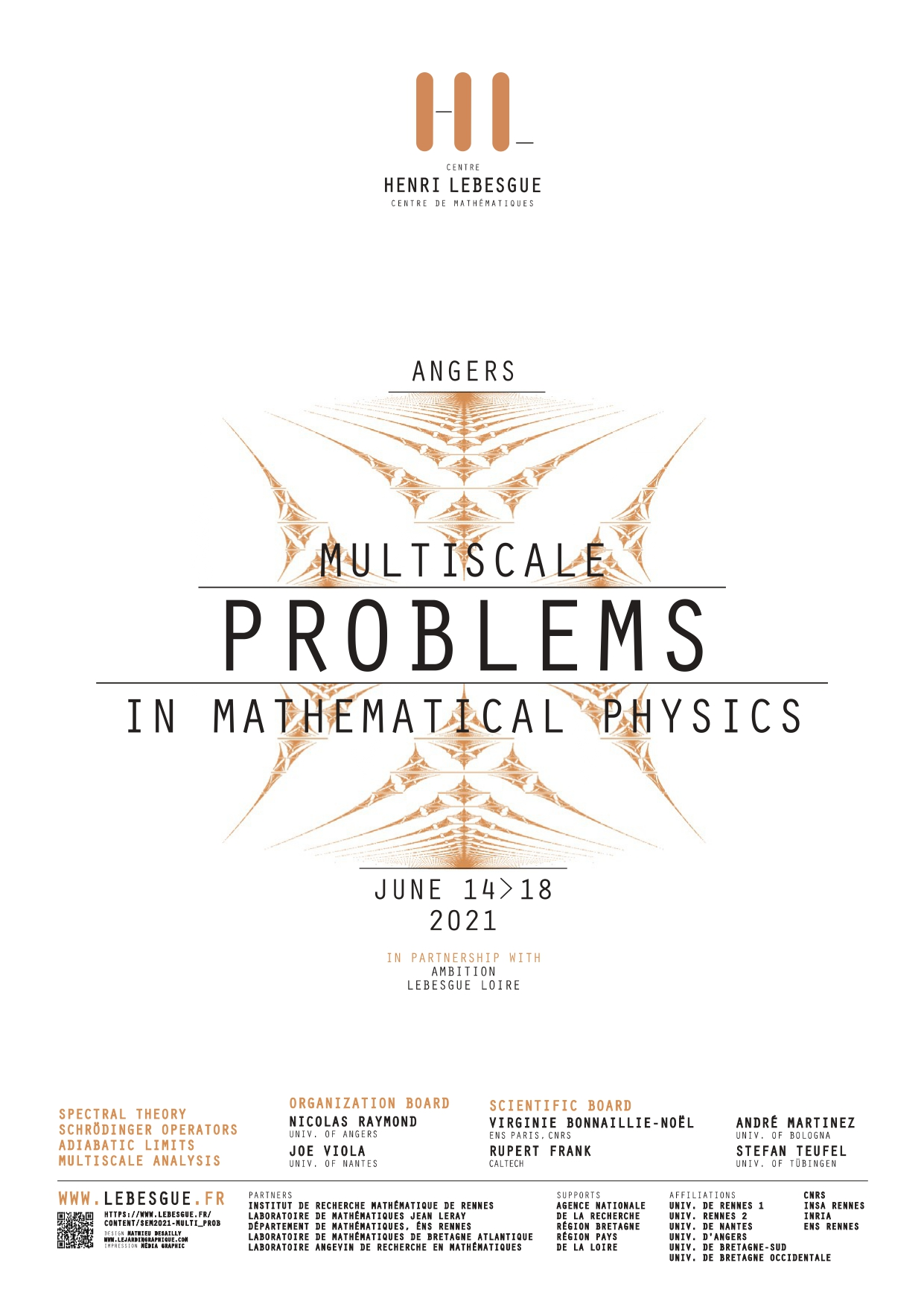placeholder

## Primary tabs

### Wednesday, June 16, 2021

 All day

Before 01
01
02
03
04
05
06
07
08
09
10
11
12
13
14
15
16
17
18
19
20
21
22
23

Conference - Multiscale problems in mathematical physics
Jun 14, 2021 to Jun 16, 2021Angers, from june 14th to june 16th 2021

Organization board: Nicolas RAYMOND, Joe VIOLA

The aim of this workshop is to present the latest advances involving multiscale phenomena arising in Physics. This will allow for the interaction between different research communities and stimulate new investigations on a large array of recent problems, from dynamical problems to spectral theory.

### Speakers

Paul Alphonse (ENS Lyon)

Victor Arnaiz (Université Paris-Saclay)

Vincent Bruneau (Université de Bordeaux)

Mattia Cafasso (University of Angers)

Éric Cancès (École des Ponts Paristech)

Clotilde Fermanian Kammerer (University Paris-Est Creteil, CNRS)

Louis Garrigue (École des Ponts)

Frédéric Hérau (University of Nantes)

Jonas Lampart (Université de Bourgogne)

Loïc Le Treust (Université d'Aix Marseille)

Léo Morin (Université de Rennes 1)

Tiến-Tài Nguyễn (Université de Paris 13)

Van Hoi Nguyen (Université de Rennes 1)

Nicolas Rougerie (ENS Lyon)

Julien Sabin (École Polytechnique)

Nir Schwartz (Université Paris-Saclay)

Nikolay Tzevtkov (University of Cery-Pontoise)

Conference - Multiscale problems in mathematical physics
Jun 16, 2021

After a brief introduction to the original Tracy-Widom distribution and its applications, I will describe some generalisations ("higher order Tracy--Widom distributions") recently introduced in the physical and mathematical literature, and give an expression of them in terms of solutions of certain equations of Painlevé type. Time permitting, I will explain how the theory of integrable ODEs allows us to study the large deviations of these distributions.

Conference - Multiscale problems in mathematical physics
Jun 16, 2021

We study Dirac operators on two-dimensional domains coupled to a magnetic field perpendicular to the plane. We focus on the infinite-mass boundary condition (also called MIT bag condition). In the case of bounded domains, we establish the asymptotic behavior of the low-lying (positive and negative) energies in the limit of strong magnetic field.

Conference - Multiscale problems in mathematical physics
Jun 16, 2021

Weyl laws provide the asymptotics of the eigenvalue counting function (as well as its pointwise density) of the discrete spectrum of some Schrödinger operators. In this talk I will explain how the introduction of a singular potential influences these asymptotics. This is a joint work with Rupert Frank (Caltech/Munich).

Conference - Multiscale problems in mathematical physics
Jun 16, 2021

I will present new results in the study of damped-wave equations for systems close to being completely integrable. Precisely, we will consider sub-principal perturbations of semiclassical harmonic oscillators and damped Baouendi-Grushin operators. For these systems, we obtain microlocal resolvent estimates near the real axis when weak-geometric control conditions are assumed. We test some of these estimates via the construction of quasimodes within the damping region.

This talk is based on joint works with Gabriel Rivière, Fabricio Macià and Chenmin Sun.

Conference - Multiscale problems in mathematical physics
Jun 16, 2021

The magnetic Laplacian is a Schrödinger operator with magnetic field. In this talk, we focus on its semi-excited spectrum in the semiclassical limit. We show how the classical cyclotron motion influence the semiclassical spectrum, and we deduce a precise description of the eigenvalues in magnetic wells.

Conference - Multiscale problems in mathematical physics
Jun 16, 2021

We will discuss the asymptotic analysis of a Schrödinger equation modeling the dynamics of an electron in a crystal for small wave-length comparable to the characteristic scale of the crystal. We will be interested in the description of the limit of time averaged energy densities for rather general initial data. We shall decompose these initial data on Bloch bands and authorize leading order contributions on modes that cross. We will explain how conical crossing of Bloch modes do not trap the energy and do not prevent dispersion despite the singularity they create. We will also describe on examples the effects of critical points of the Bloch modes localized at crossing points.